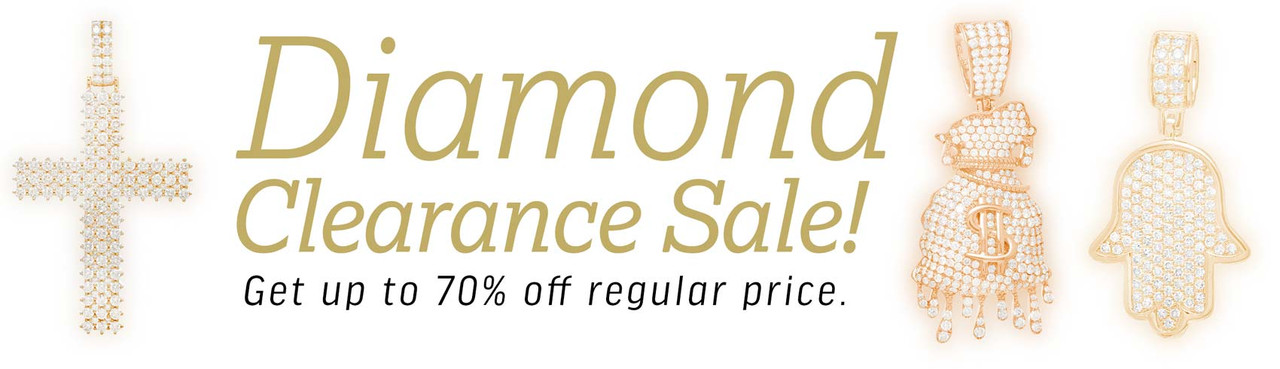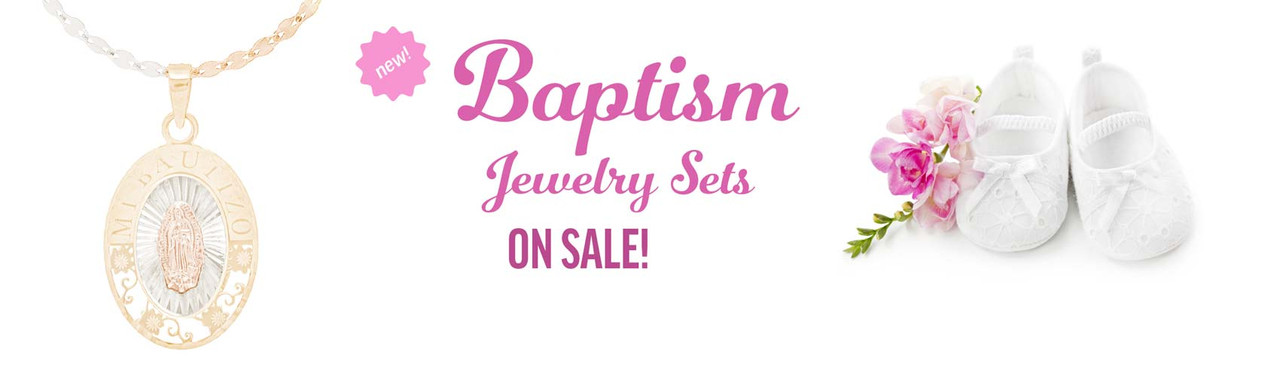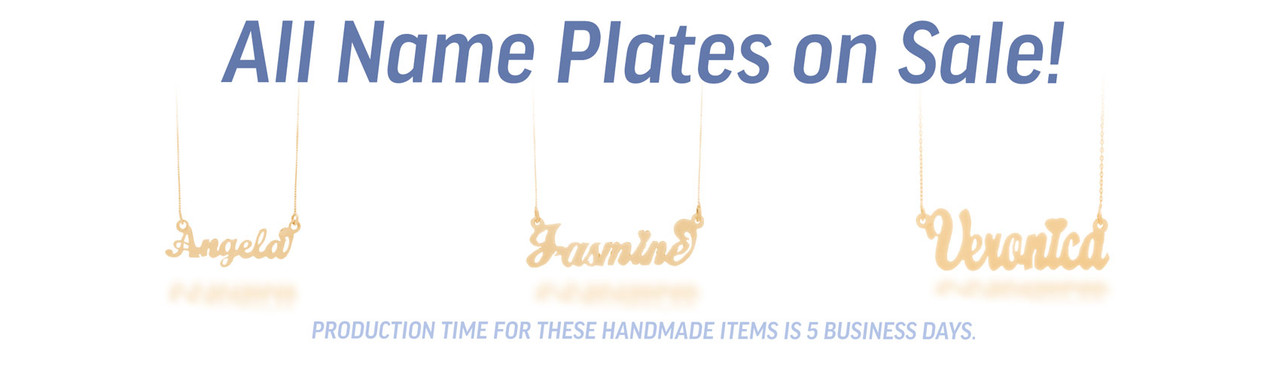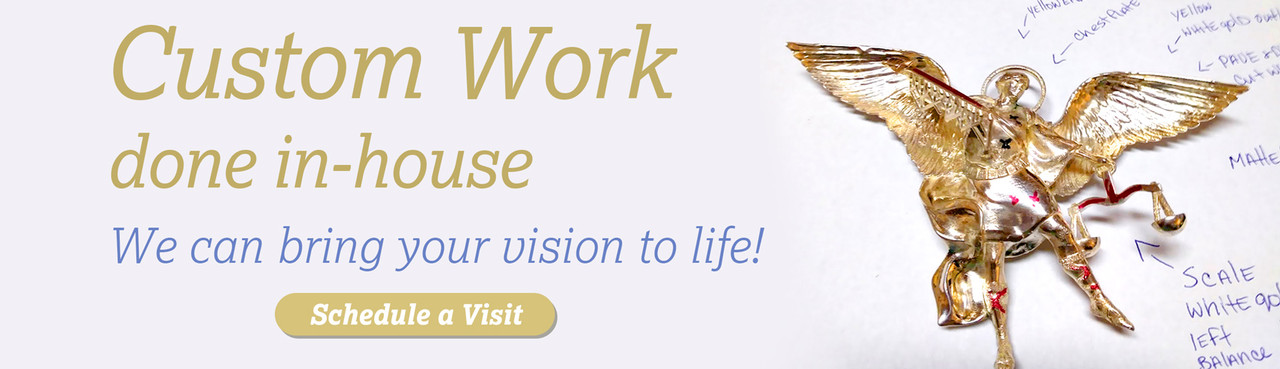Was: \$6,300.00
Now: \$3,300.00

Was: \$6,300.00
Now: \$3,300.00

Was: \$11,500.00
Now: \$4,999.00

Was: \$8,800.00
Now: \$3,990.00

Was: \$11,250.00
Now: \$4,950.00

Was: \$12,000.00
Now: \$5,000.00

Was: \$8,292.00
Now: \$1,250.00

Was: \$10,500.00
Now: \$4,999.00

Was: \$13,000.00
Now: \$5,900.00

Was: \$9,300.00
Now: \$3,500.00

Was: \$2,500.00
Now: \$990.00

Was: \$7,800.00
Now: \$3,900.00

## Most Popular

Was: \$425.00
Now: \$297.50

Was: \$470.00
Now: \$329.00

Was: \$690.00
Now: \$483.00

Was: \$290.00
Now: \$203.00

Was: \$640.00
Now: \$448.00

Was: \$1,030.00
Now: \$721.00

Was: \$464.00
Now: \$324.80

#### 1.5mm Solid Diamond Cut Rope Chain - 16", 18", 20", 22", 24"

\$345.10 - \$518.00

Was: \$560.00
Now: \$392.00

Was: \$856.00
Now: \$599.20

Was: \$160.00
Now: \$112.00

Was: \$247.00
Now: \$172.90

Was: \$11,585.00
Now: \$8,109.50

Was: \$2,132.00
Now: \$1,492.40

Was: \$290.00
Now: \$203.00

Was: \$667.00
Now: \$466.90

Was: \$640.00
Now: \$448.00

Was: \$247.00
Now: \$172.90

Was: \$2,262.00
Now: \$1,583.40

Was: \$827.00
Now: \$578.90

Was: \$247.00
Now: \$172.90